## Tamilnadu Samacheer Kalvi 6th Maths Solutions Term 1 Chapter 5 Statistics Ex 5.4

Miscellaneous Practice Problems

Question 1.
The heights (in centimetres) of 40 children are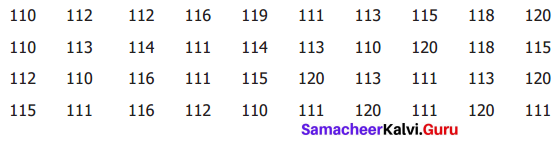Prepare a tally mark table.
Solution: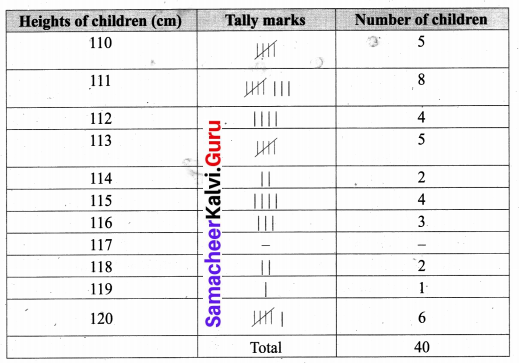Question 2.
There are 1000 students in a school. Data regarding the mode of transport of the students as given below. Draw a pictograph to represent the data.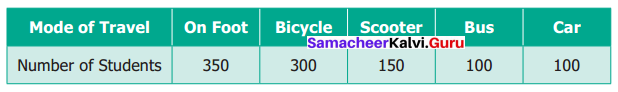Solution:
The pictograph for the mode of transport of students.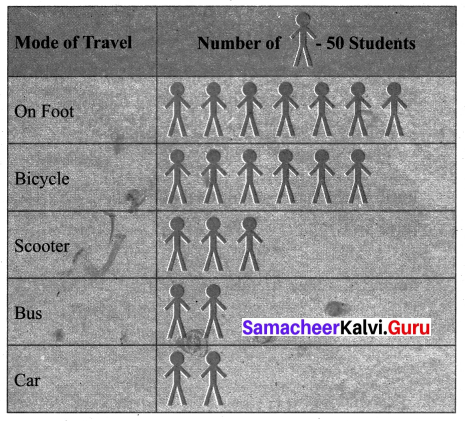Question 3.
The following pictograph shows the total savings of a group of friends in a year. Each picture represents a saving of Rs. 100. Answer the following questions.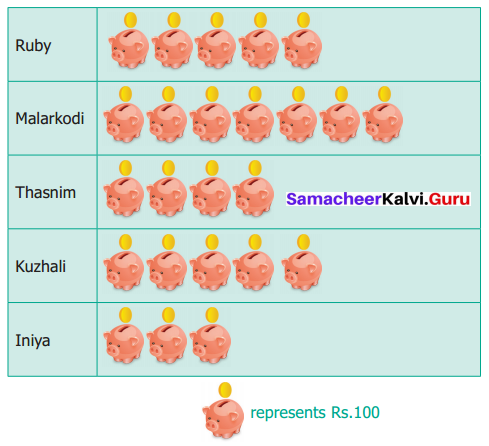(i) What is the ratio of Ruby’s saving to that of Thasnim’s?
(ii) What is the ratio of Kuzhali’s savings to that of others?
(iii) How much is Iniya’s savings?
(iv) Find the total amount of savings of all your friends?
(v) Ruby and Kuzhali save the same amount. Say True or False.
Solution:
(i) Ratio of Ruby’s saving to that of Thasnim’s
$$=\frac{\text { Ruby’s saving }}{\text { Thasnim’s saving }}=\frac{5 \times 100}{4 \times 100}=\frac{5}{4}=5: 4$$
Ratio of Ruby’s saving to that of Thasnims = 5 : 4
(ii) Ratio of Kuzhali’s savings to that of others
$$=\frac{\text { kuzhali’s saving }}{\text { others saving }}=\frac{5 \times 100}{19 \times 100}=\frac{5}{19}=5: 19$$
Ratio of Kuzhali’s saving to that of others = 5 : 19
(iii) Iniya’s saving = 3 × 100 = ₹ 300
(iv) Saving of all the friends = (5 + 7 + 4 + 5 + 3) × 100 = 24 × 100 = ₹ 2400.
Total savings = ₹ 2400
(v) True.

Challenging Problems

Question 4.
The table shows the number of moons that orbit each of the planets in our solar system.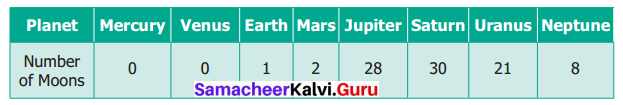Make a Bar graph for the above data.
Solution: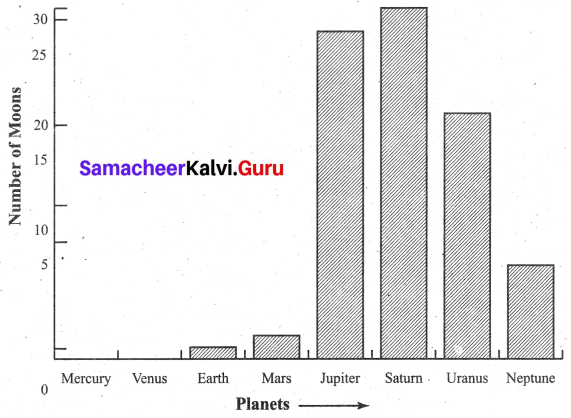Question 5.
The predictions of Weather in the month of September is given below: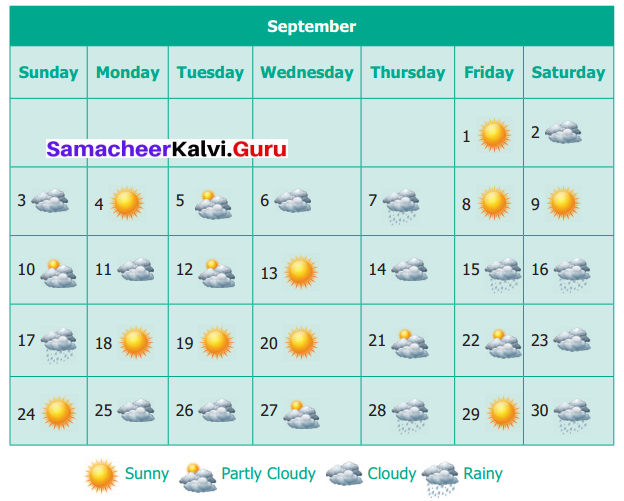(i) Make a frequency table of the types of weather by reading the calender.
(ii) How many days are either cloudy or partly cloudy
(iii) How many days do not have rain? Give two ways to find the answer?
(iv) Find the ratio of the number of sunny days to Rainy days.
Solution:
Frequency Table for the Type of weather for the month of September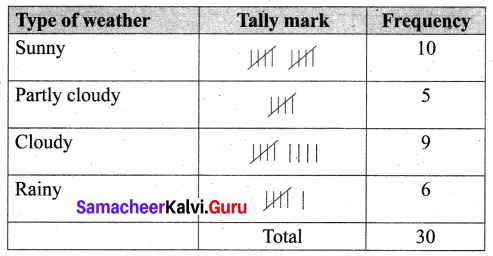(ii) 14 days.
(iii) For 24 days there is no rain.
(a) From the total 30 days, we subtract the rainy days i.e 30 – 6 = 24 days (from the frequency table)
(b) From the picture, we can count the non-rainy days.
(iv) Ratio of number of Sunny day to Rainy days
$$=\frac{\text { Number of Sunny days }}{\text { Number of Rainy days }}=\frac{10}{6}=\frac{5}{3}=5: 3$$
The ratio of a number of Sunny days to Rainy days = 5 : 3.Question 6.
26 students were interviewed to find out what they want to become in future. Their responses are given in the following table.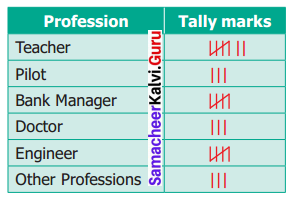Represent this data using the pictograph
Solution: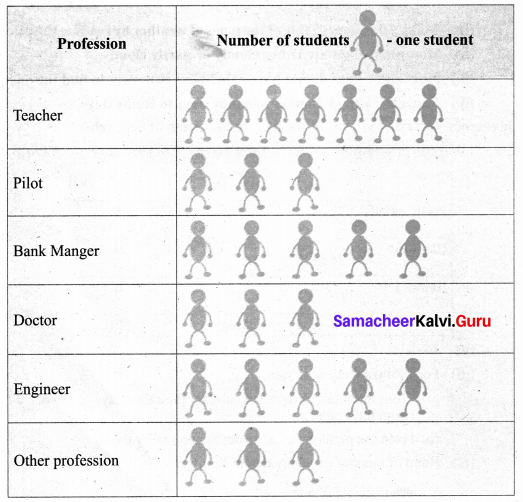Question 7.
Yasmin of class VI was given a task to count the number of books which are biographies, in her school library. The information collected by her is represented as follows.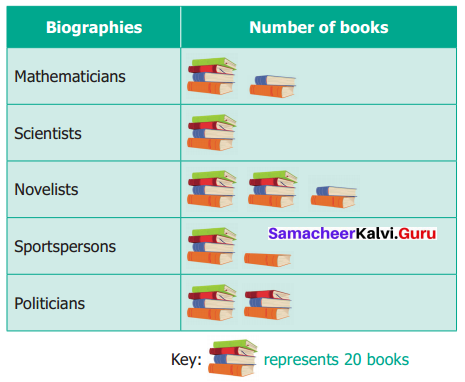Observe the pictograph and answer the following questions.
(i) Which title has the maximum number of biographies?
(ii) Which title has the minimum number of biographies?
(iii) Which title has exactly half the number of biographies as Novelists?
(iv) How many biographies are there on the title of Sportspersons?
(v) What is the total number of biographies in the library?
Solution:
(i) ‘The title Novelists’ have the maximum number of biographies
(ii) ‘The title Scientists’ have the minimum number of biographies.
(iii) ‘Sportspersons’ title has exactly half the number of biographies as Novelist.
(iv) $$(1 \times 20)+\frac{20}{4}=20+5=25$$ biographies are there in the title sportsperson.
(v) 8 × 20 = 160 biographies are there in the library.

Question 8.
The bar graph illustrates the results of a survey conducted on vehicles crossing over a Toll Plaza in on hour.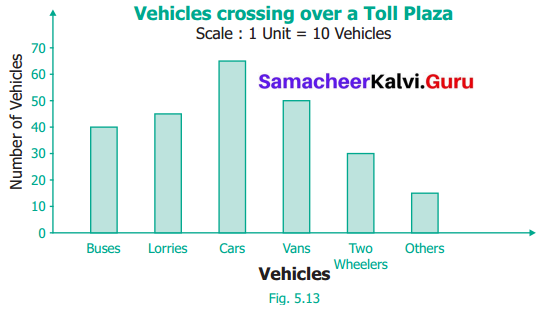Observe the bar graph carefully and fill up the following table.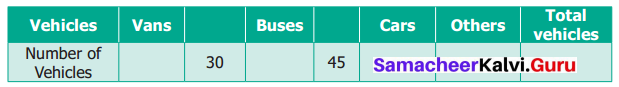Solution: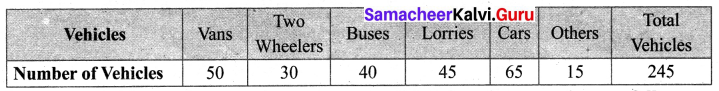Question 9.
The lengths (in the nearest centimetre) of 30 drumsticks are given as follows.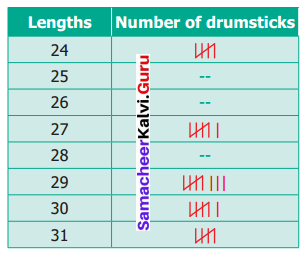Draw the bar graph showing the same information.
Solution:
The bar graph showing the same information is given below: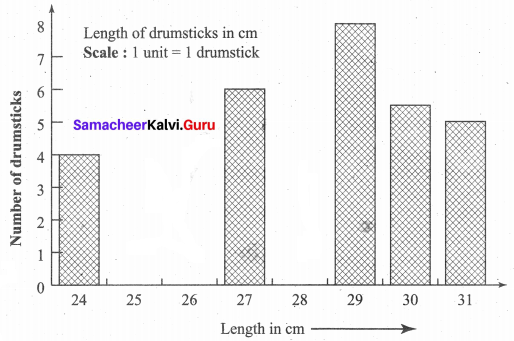Question 10.
Given two angles are supplementary i.e. their sum = 180°.
Solution:
Let the angle be x.
Then anothcf angle = x + 20 (given)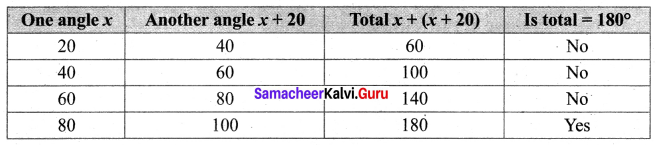The two angles are 80° and 100°